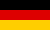# Bri's worldelectronics, programming and more

deutsch# Non-inverting Schmitt Trigger calculator

Non-inverting Schmitt Trigger calculation. First one have to enter the threshold and hysteresis voltage. The button Start is used to start the calculation.

The results will be shown in the result table below. You may use a combination of two resistors if one resistor is not good enough.

Name Description Value
V+ positive supply voltage V
V- negative supply voltage V
I cross current mA
V_out max maximum OpAmp output voltage V
V_out min minimum OpAmp output voltage V
V_th threshold voltage V
V_hys hysteresis voltage V
R1 input resistance kΩ
E series preferred values

## Result calculated

R1 R2 R3 R4 V_low V_high V_th V_hys

## Selected resistors

R1 R2 R3 R4 V_low V_high V_th V_hys